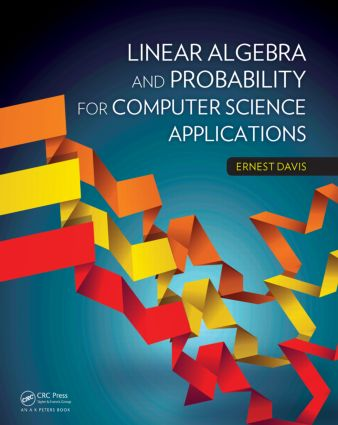# Linear Algebra and Probability for Computer Science Applications

## 1st Edition

A K Peters/CRC Press

431 pages

Hardback: 9781466501553
pub: 2012-05-02
\$72.95
x
eBook (VitalSource) : 9780429067525
pub: 2012-05-02
from \$36.48

FREE Standard Shipping!

### Description

Based on the author’s course at NYU, Linear Algebra and Probability for Computer Science Applicationsgives an introduction to two mathematical fields that are fundamental in many areas of computer science. The course and the text are addressed to students with a very weak mathematical background. Most of the chapters discuss relevant MATLAB® functions and features and give sample assignments in MATLAB; the author’s website provides the MATLAB code from the book.

After an introductory chapter on MATLAB, the text is divided into two sections. The section on linear algebra gives an introduction to the theory of vectors, matrices, and linear transformations over the reals. It includes an extensive discussion on Gaussian elimination, geometric applications, and change of basis. It also introduces the issues of numerical stability and round-off error, the discrete Fourier transform, and singular value decomposition. The section on probability presents an introduction to the basic theory of probability and numerical random variables; later chapters discuss Markov models, Monte Carlo methods, information theory, and basic statistical techniques. The focus throughout is on topics and examples that are particularly relevant to computer science applications; for example, there is an extensive discussion on the use of hidden Markov models for tagging text and a discussion of the Zipf (inverse power law) distribution.

Examples and Programming Assignments

The examples and programming assignments focus on computer science applications. The applications covered are drawn from a range of computer science areas, including computer graphics, computer vision, robotics, natural language processing, web search, machine learning, statistical analysis, game playing, graph theory, scientific computing, decision theory, coding, cryptography, network analysis, data compression, and signal processing.

Homework Problems

Comprehensive problem sections include traditional calculation exercises, thought problems such as proofs, and programming assignments that involve creating MATLAB functions.

MATLAB

Desk calculator operations

Booleans

Nonstandard numbers

Loops and conditionals

Script file

Functions

Variable scope and parameter passing

I: Linear Algebra

Vectors

Definition of vectors

Applications of vectors

Basic operations on vectors

Dot product

Vectors in MATLAB: Basic operations

Plotting vectors in MATLAB

Vectors in other programming languages

Matrices

Definition of matrices

Applications of matrices

Simple operations on matrices

Multiplying a matrix times a vector

Linear transformation

Systems of linear equations

Matrix multiplication

Vectors as matrices

Algebraic properties of matrix multiplication

Matrices in MATLAB

Vector Spaces

Subspaces

Coordinates, bases, linear independence

Orthogonal and orthonormal basis

Operations on vector spaces

Null space, image space, and rank

Systems of linear equations

Inverses

Null space and Rank in MATLAB

Vector spaces

Linear independence and bases

Sum of vector spaces

Orthogonality

Functions

Linear transformations

Inverses

Systems of linear equations

The general definition of vector spaces

Algorithms

Gaussian elimination: Examples

Gaussian elimination: Discussion

Computing a matrix inverse

Inverse and systems of equations in MATLAB

Ill-conditioned matrices

Computational complexity

Geometry

Arrows

Coordinate systems

Simple geometric calculations

Geometric transformations

Change of Basis, DFT, and SVD

Change of coordinate system

The formula for basis change

Confusion and how to avoid it

Nongeometric change of basis

Color graphics

Discrete Fourier transform (Optional)

Singular value decomposition

Further properties of the SVD

Applications of the SVD

MATLAB

II: Probability

Probability

The interpretations of probability theory

Finite sample spaces

Basic combinatorial formulas

The axioms of probability theory

Conditional probability

The likelihood interpretation

Relation between likelihood and sample space probability

Bayes’ law

Independence

Random variables

Application: Naive Bayes’ classification

Numerical Random Variables

Marginal distribution

Expected value

Decision theory

Variance and standard deviation

Random variables over infinite sets of integers

Three important discrete distributions

Continuous random variables

Two important continuous distributions

MATLAB

Markov Models

Stationary probability distribution

Hidden Markov models and the k-gram model

Confidence Intervals

The basic formula for confidence intervals

Application: Evaluating a classifier

Bayesian statistical inference (Optional)

Confidence intervals in the frequentist viewpoint: (Optional)

Hypothesis testing and statistical significance

Statistical inference and ESP

Monte Carlo Methods

Finding area

Generating distributions

Counting

Counting solutions to DNF (Optional)

Sums, expected values, integrals

Probabilistic problems

Resampling

Pseudo-random numbers

Other probabilistic algorithms

MATLAB

Information and Entropy

Information

Entropy

Conditional entropy and mutual information

Coding

Entropy of numeric and continuous random variables

The principle of maximum entropy

Statistical inference

Maximum Likelihood Estimation

Sampling

Uniform distribution

Gaussian distribution: Known variance

Gaussian distribution: Unknown variance

Least squares estimates

Principal component analysis

Applications of PCA

References

Notation

Index